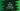# C++ program to find the centroid of a triangle# Introduction :

In this C++ programming tutorial, we will learn how to find the centroid of a triangle. The program will take the vertices as input from the user. It will find the centroid and print it out.

With this tutorial, you will learn how to read user inputs, how to print a message to the user and how to perform basic mathematical operations on C++.

## Centroid of a triangle :

The centroid of a triangle is the middle point of the triangle. For example, if the vertices of the triangle are (x1, y1), (x2, y2), (x3, y3), its centroid will be :

``````x = (x1 + x2 + x3)/3
y = (y1 + y2 + y3)/3``````

In our program, we will take the values of x and y from the user.

## C++ program :

``````#include <iostream>
using namespace std;

int main()
{
float x1, y1, x2, y2, x3, y3;
cout << "x1 : "; cin >> x1;

cout << "y1 : "; cin >> y1;

cout << "x2 : "; cin >> x2;

cout << "y2 : "; cin >> y2;

cout << "x3 : "; cin >> x3;

cout << "y3 : "; cin >> y3;

cout << "Centroid is : (" << (x1 + x2 + x3) / 3 << "," << (y1 + y2 + y3) / 3 << ")" << endl;

return 0;
}``````

## Sample Output :

``````x1 : 5
y1 : 5
x2 : 5
y2 : 4
x3 : 3
y3 : 2
Centroid is : (4.33333,3.66667)``````

### Conclusion :

We have learned how to find the centroid in C++. Try to run the above program and drop one comment below if you have any questions.